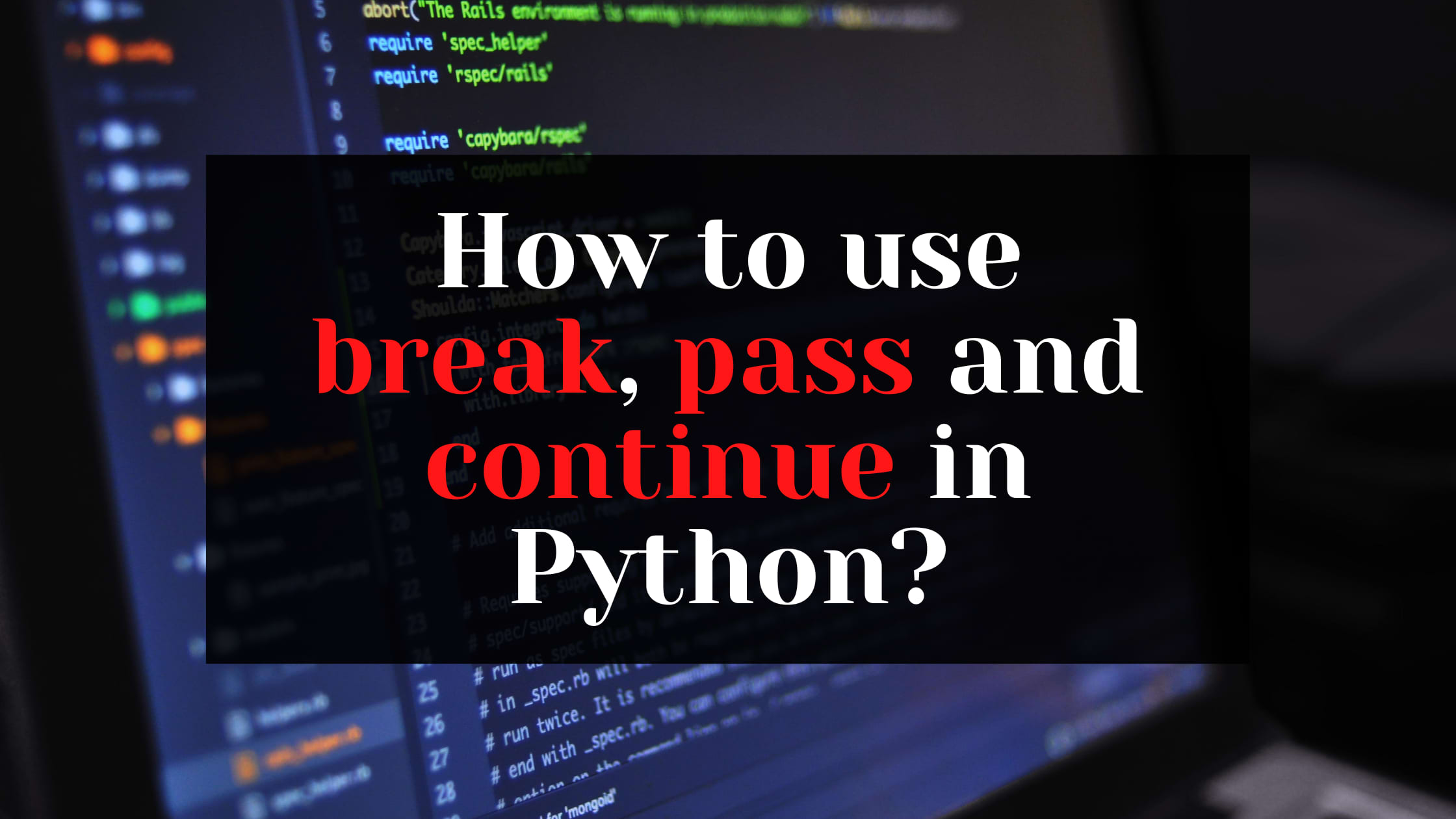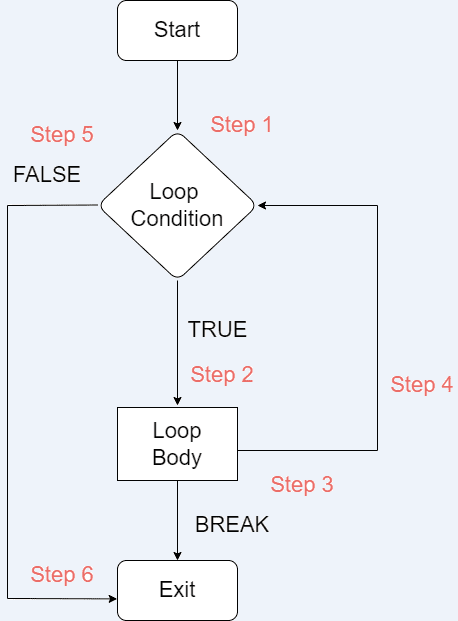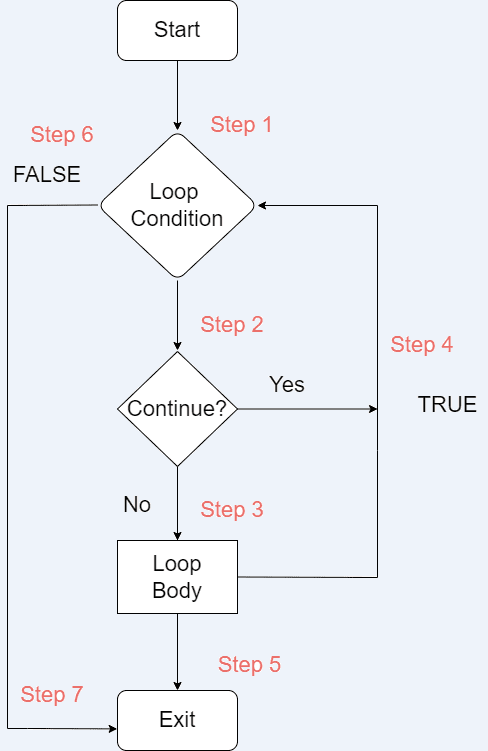# Ashutosh Writes# How to use break, pass and continue in Python?

## Introduction

Loops help you iterate over a list, tuple, dictionary, set, or string. The code inside a loop is executed multiple times until the condition fails. But sometimes, you wish to exit the loop or skip an iteration or ignore the condition. These can be achieved by the use of loop control statements.

In this tutorial, we will learn about the three loop control statements in Python with examples - `break`, `continue` and `pass`.

## Python `break` Statement

The break statement is used to terminate the loop in which it is used. In the case of nested loops, it will terminate the current loop in which it is used.

The flow chart for the break statement is as follows:The execution is as follows:

Step 1: The loop starts

Step 2: If the condition is true, the loop body is executed.

Step 3: If the loop body contains `break` statement, the control exits out of the loop and goes to Step 6.

Step 4: After the body is executed, it goes to the next iteration in Step 4.

Step 5: If the condition is false, the control exits the loop and goes to Step 6.

Step 6: The loop terminates.

Let us now look at a few examples of `break` statements:

##### Example 1
``````nums = [1, 3, 5, 16, -7, 9, 7]

for num in nums:
if num < 0:
print("I found a break statement! Bye Bye...")
break
print("Would I be printed?")
print(num)
print("Outside loop!")
``````

Can you guess the output of the above code?

``````1
3
5
16
I found a break statement! Bye Bye...
Outside loop!
``````

I hope you guessed it right! The loop executes normally till 16. As soon as it finds a number less than 0, i.e. -7, it enters the `if` condition. Inside the `if` condition, first it prints the message and then finds a `break` statement. Due to the `break` statement, it exits out of the loop, and the second `print` statement inside the `if` condition is not printed. It then finally prints the last `print` statement.

##### Example 2
``````nums = [1, 3, 5, 16, -7, 9, 7]

i = 0
while i < len(nums):
if nums[i] < 0:
print("I found a break statement! Bye Bye...")
break
print("Would I be printed?")
print(nums[i])
i += 1
print("Outside loop!")
``````

Can you guess the output this time?

``````1
3
5
16
I found a break statement! Bye Bye...
Outside loop!
``````

Well, the output remains the same this time too. It's so because we have just replaced `for` loop with `while` loop.

##### Example 3
``````for i in range(4):
for j in range(4):
if j == 2:
break
print(i, j)
``````

Can you guess the output in the above nested-loop code?

``````0 0
0 1
1 0
1 1
2 0
2 1
3 0
3 1
``````

The above code snippet uses nested for-loops. Both the loops are iterating from 0 to 4. In the second for-loop, there is a condition, wherein if the value of the second for-loop index is 2, it should break. So because of the `break` statement, the second for-loop will skip iteration for 2 and 3.

## Python `continue` Statement

Using the `continue` statement, you can skip the code after it and the control goes back to the start of the next iteration.

The flow chart for the break statement is as follows:The execution is as follows:

Step 1: The loop starts

Step 2: If the condition is true, the control enters the loop. If there is a `continue` statement, it goes to Step 4 and starts the next iteration.

Step 3: The loop body is executed.

Step 4: If there is a `continue` statement or the loop execution inside the body is done, it will go for the next iteration.

Step 5: If the loop execution is complete, the control exits the loop and goes to Step 7.

Step 6: If the loop condition is false, the control exits the loop and goes to Step 7.

Step 7: The loop terminates.

Let us now look at a few examples of `continue` statements:

##### Example 1
``````nums = [1, 3, 5, 16, -7, 9, 7]

for num in nums:
if num < 0:
print("I found a continue statement!")
continue
print("I would be skipped!")
print(num)
``````

In the above code, as soon as the control reaches -7, it enters the if condition where it first prints the print statement. Then it finds the continue statement which tells it to skip the code below it and move to the next iteration. Hence -7 will never be printed.

``````1
3
5
16
I found a continue statement!
9
7
``````
##### Example 2
``````nums = [1, 3, 5, 16, -7, 9, 7]

i = 0
while i < len(nums):
if nums[i] < 0:
print("I found a continue statement!")
i += 1
continue
print("I would be skipped!")
print(nums[i])
i += 1
``````

The output for the above snippet would remain the same. Notice the two `i += 1` statements. The second one is the usual one. The first one, inside the `if` condition, helps us avoid an infinite loop.

``````1
3
5
16
I found a continue statement!
9
7
``````
##### Example 3
``````for i in range(4):
for j in range(4):
if j == 2:
continue
print(i, j)
``````

The above code snippet uses nested for-loops. Both the loops are iterating from 0 to 4. In the second for-loop, there is a condition, wherein if the value of the second for-loop index is 2, it should continue. So because of the `continue` statement, the second for-loop will skip iteration for 2 and go for 3.

``````0 0
0 1
0 3
1 0
1 1
1 3
2 0
2 1
2 3
3 0
3 1
3 3
``````

## Python pass Statement

This statement is used as a placeholder within Python loops, functions, classes, or conditions to tell the user that this will be implemented later. It is a null statement and the interpreter ignores it whenever it is encountered.

Let us see examples of `pass` statement:

##### Example 1: Function
``````def my_func():
print("Hello World")
pass

my_func()
``````

Output:

``````Hello World
``````

The `pass` statement will be executed whenever the function `my_func` is called. However, in this case, it is not required to add `pass` statement.

##### Example 2: Class
``````class MyClass:
pass
``````

If you need to create a class and you're going to implement it later, you can simply add a `pass` statement to avoid the `IndentationError`.

##### Example 3: Loop
``````nums = [1, 3, 5, 16, -7, 9, 7]

for num in nums:
if num < 0:
print("I found a pass statement!")
pass
print(num)
``````

In the above snippet, the `pass` statement does nothing at all.

``````1
3
5
16
I found a pass statement!
-7
9
7
``````
##### Example 4: If Condition
``````num =3

if num == 3:
print("Before pass")
pass
print("After pass")
``````

What do you expect in this case? Do you think "After pass" will be printed?

``````Before pass
After pass
``````

Of course, it will be! The `pass` statement doesn't do anything at all.

## When to choose `break` and `continue`?

Whenever you want to terminate the loop completely, you should choose the `break` statement. However, if you wish to skip the current iteration of the loop and proceed to the next one, choosing the `continue` statement would make sense.

## Conclusion

In this tutorial, we covered most of the things around `break`, `continue` and `pass` statements in Python. We also saw various examples to have a clear understanding of how they actually work. In the end, we also learned when to choose `break` and `continue` statements.

I hope you found the tutorial helpful. Share it with your Python buddies!